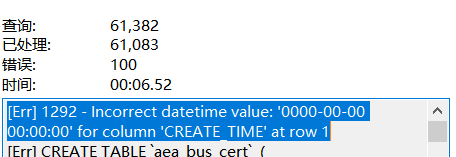C语言 插入排序 Insert Sort

``````#include <stdio.h>

void exchange(int* array, int p1, int p2)
{
if (p1 == p2)
return;
int temp = array[p1];
array[p1] = array[p2];
array[p2] = temp;
}

void insertSort(int* array, int len)
{
int sorted = 0; //the 1st data we think was already sorted
int cur;
for (cur = 1; cur < len; cur++)//start from 2nd data
{
//loop with sorted range
int sort;
for (sort = 0; sort <= sorted; sort++)
{
if (array[cur] <= array[sort])
{
// let current data move forward one by one and stop at right postion
int curPos = cur;
while (curPos != sort)
{
exchange(array, curPos, curPos - 1);
curPos--;
}
break;
}
}
sorted++;
}
}

//From Intruduction Of Alogrithim
void insertSort1(int* array, int len)
{
for (int i = 1; i < len; i++)//loop start from 2nd data cause we think 1st is already sorted
{
int key = array[i];//current data we call it key
int j = i - 1;//watch pre data of key
while (j >= 0 && array[j] > key)
{
array[j+1] = array[j];//if pre data bigger, move to right
j--;//if pre data stiill bigger than key, move to right
}//end while for moving
array[j + 1] = key;// when move over the postion `j+1` was empty insert the key
}
}

void main()
{
intarray = { 1, 8, 3, 6, 2, 4, 7, 5, 9, 0 };
printf("before:");
int i;
for (i = 0; i <= sizeof(array) - 1; i++)
{
printf("%d ", array[i]);
}
printf("\n");
insertSort(array, sizeof(array));
printf("\n after:");
for (i = 0; i <= sizeof(array) - 1; i++)
{
printf("%d ", array[i]);
}
return;
}
``````blmius
1年前
MySQL:[Err] 1292 - Incorrect datetime value: ‘0000-00-00 00:00:00‘ for column ‘CREATE_TIME‘ at row 1Jacquelyn38
2年前
2020年前端实用代码段，为你的工作保驾护航Wesley13
1年前
java将前端的json数组字符串转换为列表helloworld_34035044
11个月前

​在我们用皕杰报表工具设计填报报表时，如何在新增行里自动增加id呢？能新增整数排序id吗？目前可以在新增行里自动增加id，但只能用uuid函数增加UUID编码，不能新增整数排序id。uuid函数说明：获取一个UUID，可以在填报表中用来创建数据ID语法：uuid()或uuid(sep)参数说明：sep布尔值，生成的uuid中是否包含分隔符''，缺省为Wesley13
1年前
Java获得今日零时零分零秒的时间（Date型）
publicDatezeroTime()throwsParseException{    DatetimenewDate();    SimpleDateFormatsimpnewSimpleDateFormat("yyyyMMdd00:00:00");    SimpleDateFormatsimp2newSWesley13
1年前
mysql设置时区
mysql设置时区mysql\_query("SETtime\_zone'8:00'")ordie('时区设置失败，请联系管理员！');中国在东8区所以加8方法二：selectcount(user\_id)asdevice,CONVERT\_TZ(FROM\_UNIXTIME(reg\_time),'08:00','0Wesley13
1年前
00：Java简单了解Stella981
1年前Wesley13
1年前
00_设计模式之语言选择Wesley13
1年前
MySQL部分从库上面因为大量的临时表tmp_table造成慢查询Stella981
Lv1

1.7w

32

26